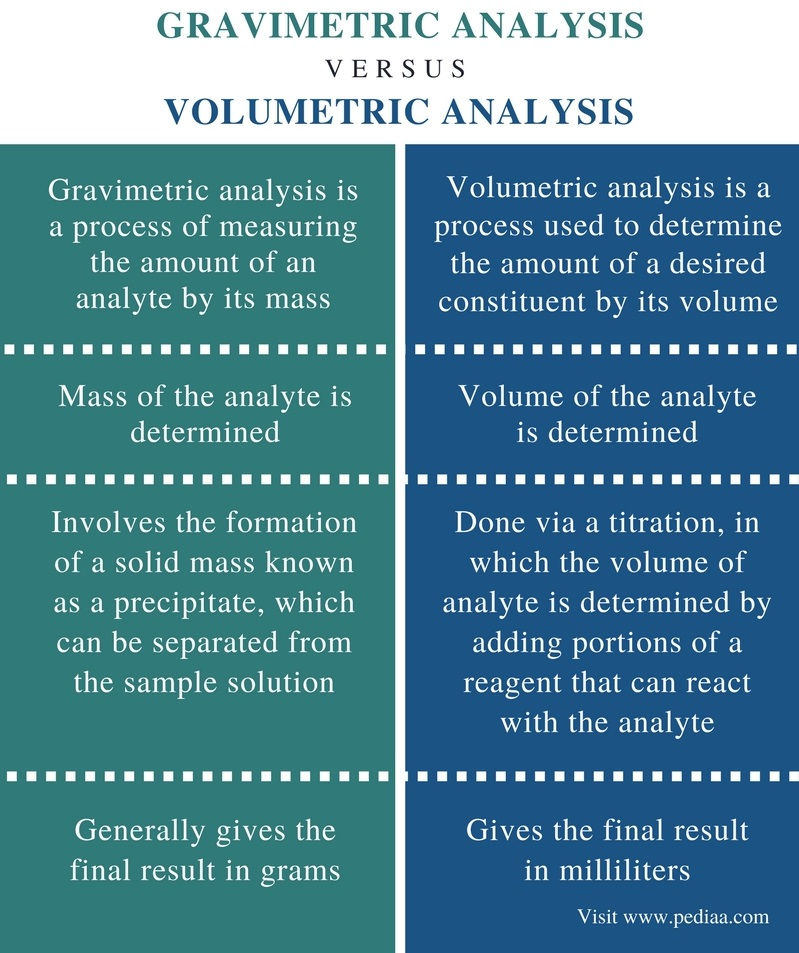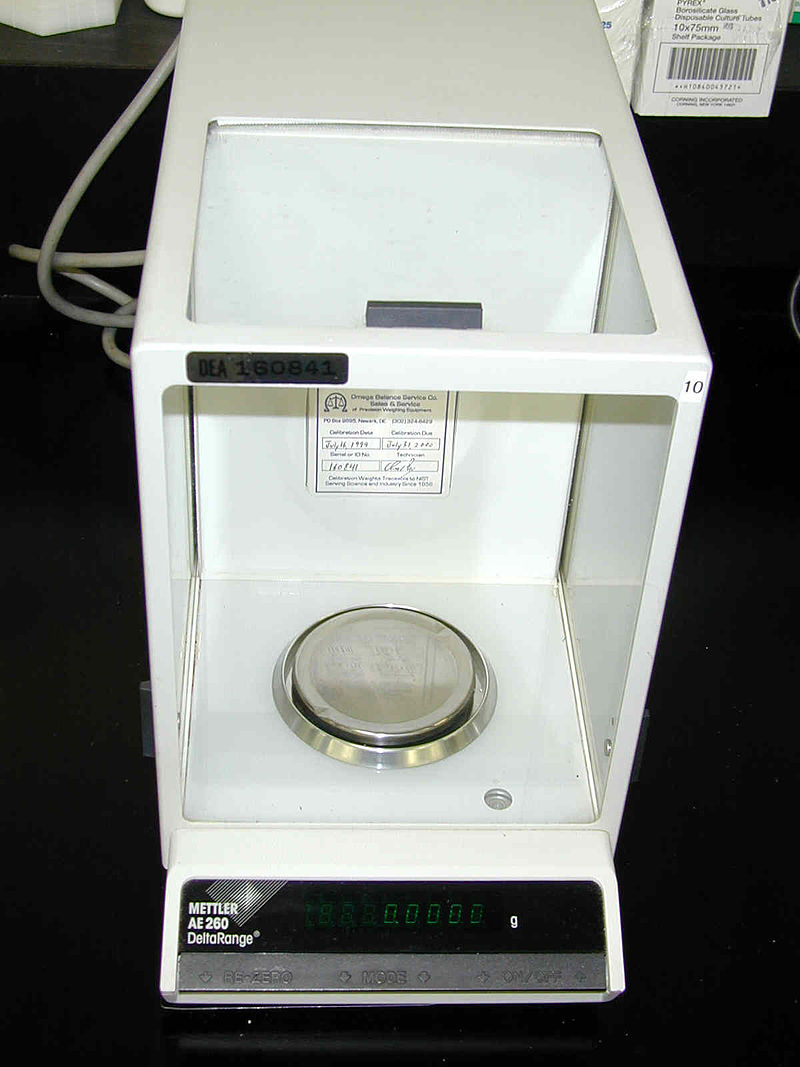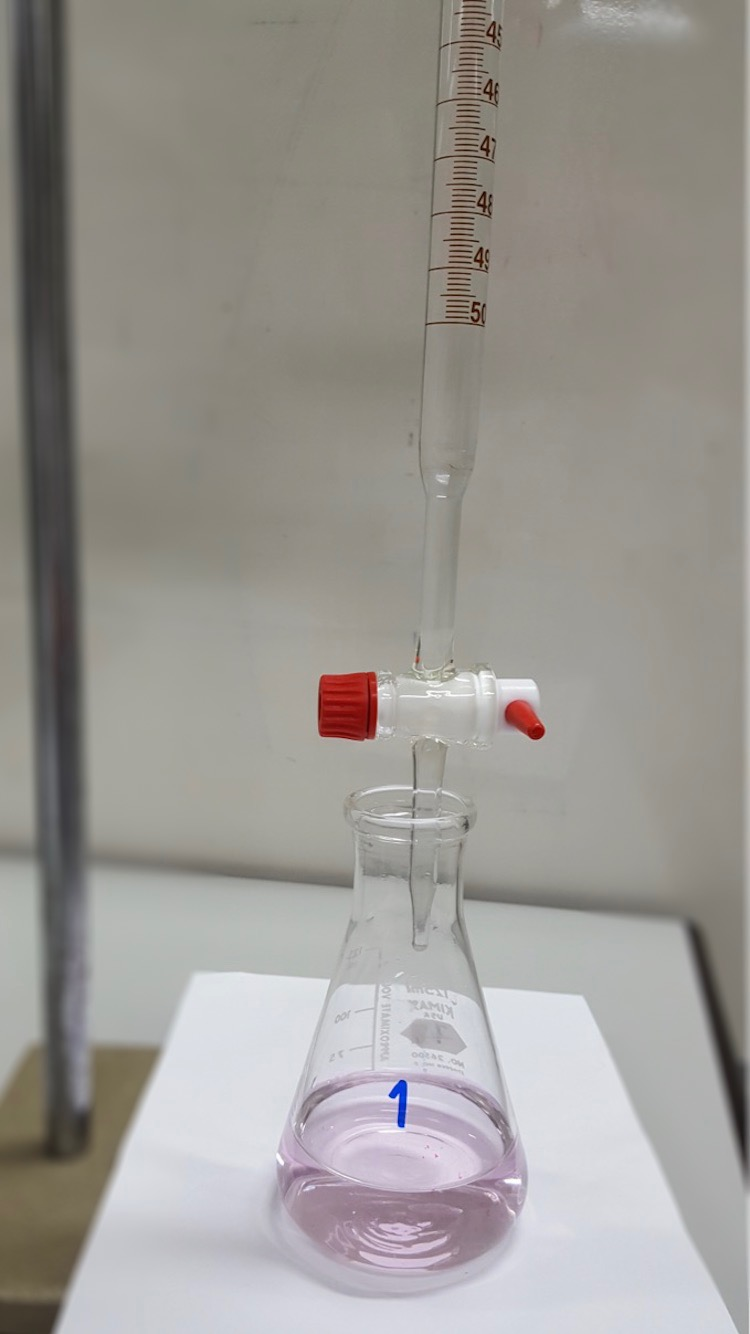# Difference Between Gravimetric and Volumetric Analysis

## Main Difference – Gravimetric vs Volumetric Analysis

The amount of a constituent present in a mixture of constituents can be determined using either gravimetric analysis or volumetric analysis. These methods can be used to determine the purity of a constituent in a given sample. The main difference between gravimetric and volumetric analysis is that in gravimetric analysis, the mass of the analyte is determined whereas, in volumetric analysis, the volume of the analyte is determined.

### Key Areas Covered

1. What is Gravimetric Analysis
– Definition, Process, Requirements
2. What is Volumetric Analysis
– Definition, Titration as a Volumetric Analysis Method
3. What is the Difference Between Gravimetric and Volumetric Analysis
– Comparison of Key Differences

Key Terms: Buchner Funnel, Filtration, Gravimetric Analysis, Mass, Titration, Volatilization, Volumetric Analysis## What is Gravimetric Analysis

Gravimetric analysis is a process of measuring the amount of an analyte by its mass. Hence, it is a quantitative determination. Here, the desired constituent in a liquid is converted to a solid form that can easily be separated and weighed in order to determine the amount of it and that solid mass can be used for further analysis. The typical gravimetric analysis will have the following steps.

1. Preparation of a solution
2. Separation of the desired constituent from the solution
3. Weighing the amount of constituent

In order to make this analysis a success, the constituent must be completely precipitated as a pure compound and it also must be easy to separate the precipitate via filtration. For the formation of a precipitate, a reagent is added to the solution. This reagent is known as a precipitating agent. Either a filtration method or a volatilization method can be used to separate the precipitate from the solution,Figure 1: An analytical balance, which can be used to get an accurate weight for a very little amount of sample obtained via precipitation.

Here, filtration includes filtering of the liquid phase, leaving the solid precipitate on the filter paper. The separation of the precipitate can be done using the following methods;

1. Filtration under gravity
2. Filtration in a Buchner funnel (vacuum filtration)
3. Volatilization of the liquid phase leaving the precipitate

Some applications of gravimetric analysis include precipitation of Ag+, Pb+2, and Hg22+ as halides, precipitation of Ca2+ as calcium oxalate (CaC2O4), precipitation of Ba2+ as Barium sulfate (BaSO4), etc.

## What is Volumetric Analysis

Volumetric analysis is a process used to determine the amount of the desired constituent by its volume. Hence it is a quantitative determination. Here, the volume of the constituent is measured via a titration (titrimetric analysis).

In titrations, a reagent that can react with the analyte is added as proportions until all analyte molecules react with the reagent molecules. When both the sample and the reagent are colorless solutions, an indicator should be used in order to determine the endpoint of the reaction. The endpoint indicates the end of the analyte molecules.Figure 2: Apparatus for Titration

The indicator is a reagent that can give a color change when the endpoint has been reached. The color change occurs when a change in the reaction mixture has occurred. For example, an acid-base indicator is sensitive to the change of pH in the reaction mixture. It gives the color change when the pH is changed. But some reagents act as self-indicators. Ex: Potassium permanganate.

The reagent used to determine the amount of analyte should completely and quickly react with all analyte molecules. The concentration of this reagent should be known and the portions added should be noted, because these details are required for the calculation of the concentration of the analyte.

## Difference Between Gravimetric and Volumetric Analysis

### Definition

Gravimetric Analysis:  Gravimetric analysis is a process of measuring the amount of an analyte by its mass.

Volumetric Analysis: Volumetric analysis is a process used to determine the amount of a desired constituent by its volume.

### Parameter Determined

Gravimetric Analysis:  In gravimetric analysis, the mass of the analyte is determined.

Volumetric Analysis: In volumetric analysis, the volume of the analyte is determined.

### Process

Gravimetric Analysis:  Gravimetric analysis involves the formation of a solid mass known as a precipitate, which can be separated from the sample solution.

Volumetric Analysis:  Volumetric analysis is done via a titration, in which the volume of analyte is determined by adding portions of a reagent (of known concentration) that can react with the analyte.

### Unit

Gravimetric Analysis:  Gravimetric analysis generally gives the final result in grams – g (sometimes in milligrams – mg).

Volumetric Analysis:  Volumetric analysis gives the final result in milliliters –mL (sometimes in microliters – μm).

### Conclusion

Gravimetric and volumetric analysis techniques are two types of analytical techniques that are used to measure the amount of a certain constituent present in a given sample.  The main difference between gravimetric and volumetric analysis is that in gravimetric analysis, the mass of the analyte is determined whereas, in volumetric analysis, the volume of the analyte is determined.

##### Reference:

1. “Volumetric Analysis.” [email protected], Available here.
2.  “Gravimetric analysis.” Encyclopædia Britannica, Encyclopædia Britannica, inc., 9 Mar. 2016, Available here.
3. “Wired Chemist.” Volumetric Analysis, Available here.

##### Image Courtesy:

1. “Analytical balance mettler ae-260” By US DEA -(Public Domain) via Commons Wikimedia
2. “Acid and Base Titration” By Kengksn – Own work (CC BY-SA 4.0) via Commons Wikimedia